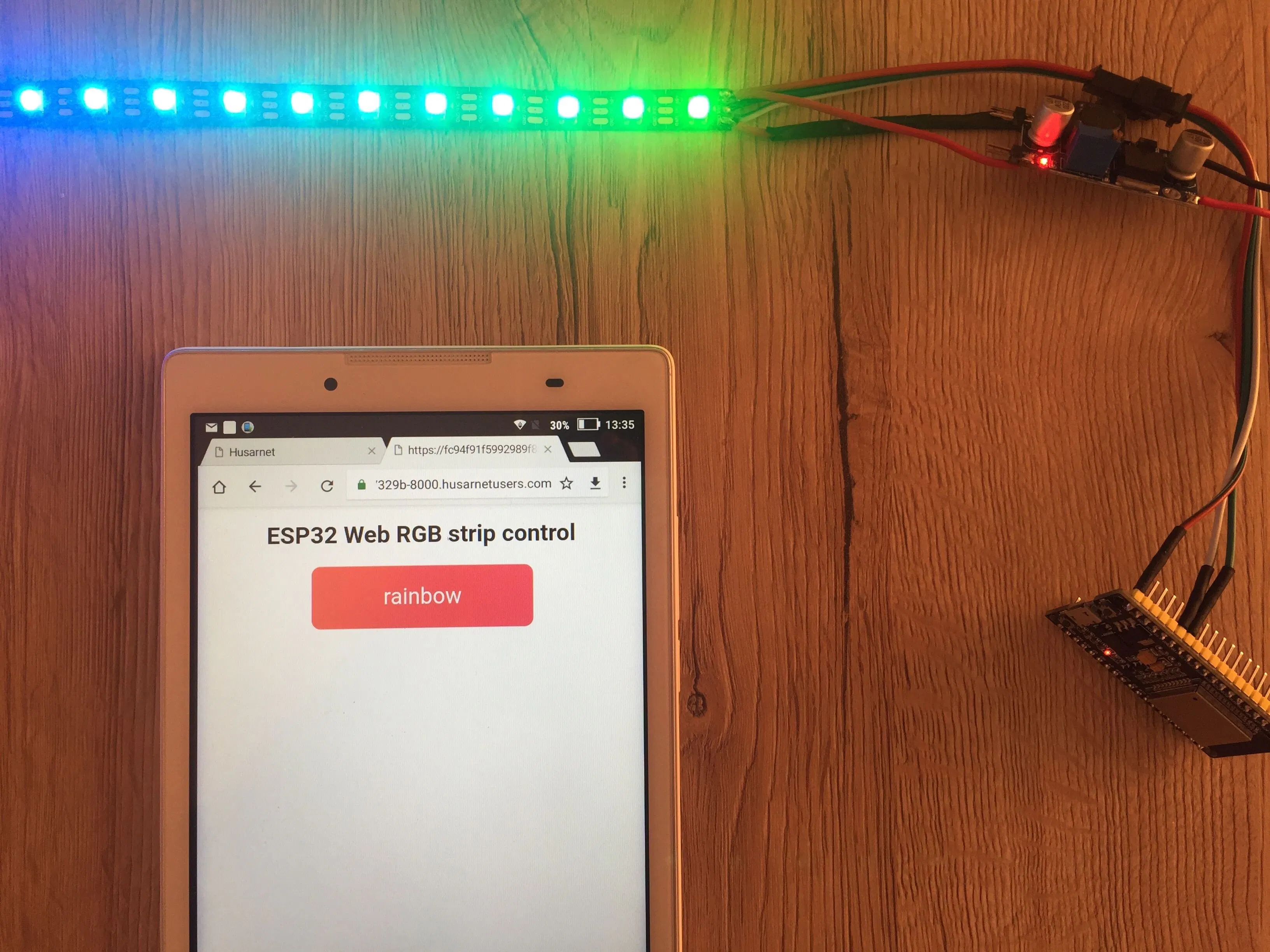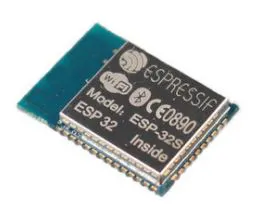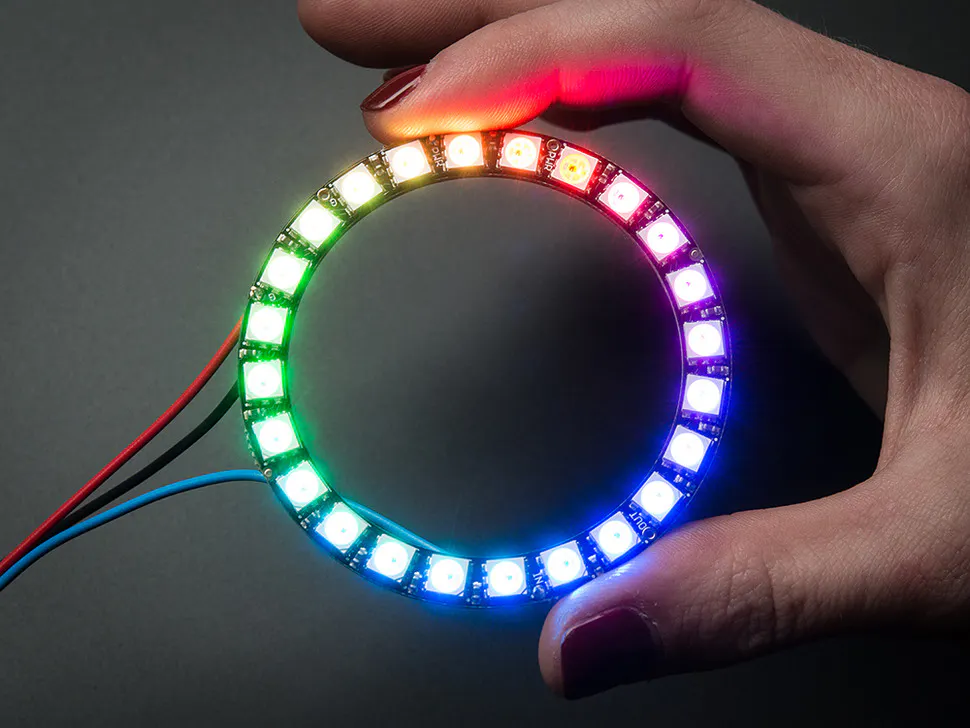Published © GPL3+

# Internet Controlled LED Strip Using ESP32 + Arduino

Control an RGB LED strip using ESP32 hosting an HTTP server written in Arduino framework. Works in local network and through the internet.

BeginnerFull instructions provided3 hours5,728## Things used in this project

### Hardware componentsEspressif ESP32S
×1Adafruit NeoPixel Ring: WS2812 5050 RGB LED
×1
 5V DC power supply
×1

### Software apps and online servicesArduino IDEHusarnet client

Read more

## Code

### ESP32_ledstrip_webserver.ino

Arduino
```#include <WiFi.h>
#include <NeoPixelBus.h>
#include <Husarnet.h>

// Which pin on the Arduino is connected to the NeoPixels?
#define PIN            12

// How many NeoPixels are attached to the Arduino?
#define NUMPIXELS      60

NeoPixelBus<NeoGrbFeature, Neo800KbpsMethod> strip(NUMPIXELS, PIN);

uint8_t red = 0;
uint8_t green = 0;
uint8_t blue = 0;

int j = 0;

#define NUM_NETWORKS 4

// Add your networks credentials here
const char* ssidTab[NUM_NETWORKS] = {
"wifi-network-1",
"wifi-network-2",
"wifi-network-3",
"wifi-network-4"
};
const char* passwordTab[NUM_NETWORKS] = {
"wifi-pass-1",
"wifi-pass-2",
"wifi-pass-3",
"wifi-pass-4"
};

HusarnetServer server(8000);

String header;

void setup() {
Serial.begin(115200);

strip.Begin();
strip.Show();

bool connectedSuccess = 0;
for (int i = 0; i < NUM_NETWORKS; i++) {
Serial.print("Connecting to ");
Serial.println(ssidTab[i]);
WiFi.begin(ssidTab[i], passwordTab[i]);
for (int j = 0; j < 10; j++) {
if (WiFi.status() != WL_CONNECTED) {
delay(500);
Serial.print(".");
} else {
connectedSuccess = true;
}
}
Serial.println("");
if (connectedSuccess == true) {
break;
}
}
if (connectedSuccess == false) {
Serial.println("WiFi network unreachable");
while (1) {
;
}
}

Serial.println("");
Serial.println("WiFi connected.");
Serial.println("IP address: ");
Serial.println(WiFi.localIP());

Husarnet.start();

server.begin();

xTaskCreate(
taskLED,          /* Task function. */
"taskLED",        /* String with name of task. */
10000,            /* Stack size in bytes. */
NULL,             /* Parameter passed as input of the task */
2,                /* Priority of the task. */
NULL);            /* Task handle. */

xTaskCreate(
taskWifi,          /* Task function. */
"taskWifi",        /* String with name of task. */
10000,            /* Stack size in bytes. */
NULL,             /* Parameter passed as input of the task */
1,                /* Priority of the task. */
NULL);            /* Task handle. */
}

void rainbow(int j) {
int k = 0;
int l = 0;
int m = 0;

for (int i = 0; i < NUMPIXELS; i++) {
if ( ( i >= 0 ) && ( i < NUMPIXELS * 1 / 3 ) ) {
red = 255 * k / (NUMPIXELS / 3);
green = 0;
blue = 255 - (255 * k / (NUMPIXELS / 3) );
k++;
}
if ( ( i >= NUMPIXELS * 1 / 3 ) && ( i < NUMPIXELS * 2 / 3 ) ) {
red = 255 - (255 * l / (NUMPIXELS / 3) );
green = 255 * l / (NUMPIXELS / 3);
blue = 0;
l++;
}
if ( ( i >= NUMPIXELS * 2 / 3 ) && ( i < NUMPIXELS * 3 / 3 ) ) {
red = 0;
green = 255 - (255 * m / (NUMPIXELS / 3) );
blue = 255 * m / (NUMPIXELS / 3);
m++;
}

strip.SetPixelColor((i + j) % NUMPIXELS, RgbColor(red, green, blue));
}

strip.Show();
delay(50);
}

void white_shine(int j) {
int k = 0;
int l = 0;
int m = 0;

for (int i = 0; i < NUMPIXELS; i++) {
if ( ( i >= 0 ) && ( i < NUMPIXELS * 1 / 3 ) ) {
red = 255 * k / (NUMPIXELS / 3);
green = 255 * k / (NUMPIXELS / 3);
blue = 255 * k / (NUMPIXELS / 3);
k++;
}
if ( ( i >= NUMPIXELS * 1 / 3 ) && ( i < NUMPIXELS * 2 / 3 ) ) {
red = 255 - (255 * l / (NUMPIXELS / 3) );
green = 255 - (255 * l / (NUMPIXELS / 3) );
blue = 255 - (255 * l / (NUMPIXELS / 3) );
l++;
}
if ( ( i >= NUMPIXELS * 2 / 3 ) && ( i < NUMPIXELS * 3 / 3 ) ) {
red = 0;
green = 0;
blue = 0;
m++;
}
Serial.printf("\r\n[R,G,B] = [%d,%d,%d]", red, green, blue);
strip.SetPixelColor((i + j) % NUMPIXELS, RgbColor(red, green, blue));
}
strip.Show();
delay(40);
}

void red_shine(int j) {
int k = 0;
int l = 0;
int m = 0;

for (int i = 0; i < NUMPIXELS; i++) {
if ( ( i >= 0 ) && ( i < NUMPIXELS * 1 / 3 ) ) {
red = 255;
green = 255 - 255 * k / (NUMPIXELS / 3);
blue = 255 - 255 * k / (NUMPIXELS / 3);
k++;
}
if ( ( i >= NUMPIXELS * 1 / 3 ) && ( i < NUMPIXELS * 2 / 3 ) ) {
red = 255;
green = (255 * l / (NUMPIXELS / 3) );
blue = (255 * l / (NUMPIXELS / 3) );
l++;
}
if ( ( i >= NUMPIXELS * 2 / 3 ) && ( i < NUMPIXELS * 3 / 3 ) ) {
red = 255;
green = 255;
blue = 255;
m++;
}
strip.SetPixelColor((i + j) % NUMPIXELS, RgbColor(red, green, blue));
}
strip.Show();
delay(40);
}

void led_white()
{
for (int i = 0; i < NUMPIXELS; i++) {
red = 255;
green = 255;
blue = 255;
strip.SetPixelColor((i + j) % NUMPIXELS, RgbColor(red, green, blue));
}
delay(5);
strip.Show();
}

void led_off() {
for (int i = 0; i < NUMPIXELS; i++) {
red = 0;
green = 0;
blue = 0;
strip.SetPixelColor((i + j) % NUMPIXELS, RgbColor(red, green, blue));
}
delay(100);
strip.Show();
}

char* getModeName(uint8_t modeNo) {
switch (modeNo) {
case 0:
return "rainbow";
case 1:
return "white shine";
case 2:
return "red shine";
case 3:
return "only white";
case 4:
return "off";
}
return "error";
}

uint8_t modeRGB = 0;
void taskLED( void * parameter )
{
while (1) {
switch (modeRGB) {
case 0:
rainbow(j++);
break;
case 1:
white_shine(j++);
break;
case 2:
red_shine(j++);
break;
case 3:
led_white();
break;
case 4:
led_off();
break;
default:
rainbow(j++);
break;
}
if (j >= NUMPIXELS) {
j = 0;
}
}

Serial.println("Ending task LED");
vTaskDelete( NULL );
}

void loop() {
while (1) {
delay(1000);
}
}

static const char* htmlHead = R"rawText(
<head>
<meta name="viewport" content="width=device-width, initial-scale=1">
<link rel="icon" href="data:,">
<style>
html {
font-family: Helvetica;
display: inline-block;
margin: 0px auto;
text-align: center;
}

.button {
background:
linear-gradient(to right, #FB6060 0%, #EC305D 100%);
border: none;
text-decoration: none;
margin: 2px;
padding: 25px 25px;
width: 300px;
border-radius: 12px;
color: white;
font-size: 30px;
cursor: pointer;
}
</style>
</head>
)rawText";

void taskWifi( void * parameter ) {

while (1) {
HusarnetClient client = server.available();

if (client) {
Serial.println("New Client.");
String currentLine = "";
Serial.printf("connected: %d\n", (int)client.connected());
while (client.connected()) {

if (client.available()) {
char c = client.read();
//          Serial.write(c);
header += c;
if (c == '\n') {
if (currentLine.length() == 0) {
client.println("HTTP/1.1 200 OK");
client.println("Content-type:text/html");
client.println("Connection: close");
client.println();

if (header.indexOf("GET /0") >= 0) {
modeRGB = 1;
}
if (header.indexOf("GET /1") >= 0) {
modeRGB = 2;
}
if (header.indexOf("GET /2") >= 0) {
modeRGB = 3;
}
if (header.indexOf("GET /3") >= 0) {
modeRGB = 4;
}
if (header.indexOf("GET /4") >= 0) {
modeRGB = 0;
}

// Head
client.println("<!DOCTYPE html><html>");
client.println(htmlHead);
// Body
client.println("<body><h1>ESP32 Web RGB strip control</h1>");
client.print("<p><a href=\"/");
client.print(modeRGB);
client.print("\"><button class=\"button\">");
client.print(getModeName(modeRGB));
client.println("</button></a></p>");
client.println("</body></html>");

client.println();
break;
} else {
currentLine = "";
}
} else if (c != '\r') {
currentLine += c;
}
}
}

header = "";

client.stop();
Serial.println("Client disconnected.");
Serial.println("");
}
}
}
```

## Credits

### Dominik

9 projects • 33 followers